Contents

# Making Simple Geometrical Shapes on Python using NumPy and Matplotlib

I might stop to write new blogs in this site so please visit dataqoil.com for more awesome blogs about computer vision projects.

Now on this series of task I am going to tackle some of interesting image processing concepts from scratch using Python and then will compare it with popular OpenCV framework. Last time I did Convolution operation from Scratch and RGB to GrayScale conversion, etc. Now is the time to draw circle, rectangle, ellipse and get the flashback of childhood. I am highly inspired by the book named Image Operators: Image Processing in Python by Jason M. Kinser. In fact I am going to use some simple geometrical concepts to draw these basic shapes using only NumPy and Matplotlib.

Also I have to mention the awesome book named The Journey of X: A Guided Tour of Mathematics by Steven Strogatz. Author really have a great way of describing the mathematical terms and I have learned lot of concepts on Mathematics from there. And author also introduced to the awesome book named The House Keeper and the Professor.

The method I am including here will be added to the previous Image Processing Class (which is also given below) I have used to do Convolution and Colorspace changes. So it will be helpful to view that one also.

## What will I do here?

• Using primary grade mathematics, I will create a simple methods to draw/create a simple geometric shapes and compare them with OpenCV’s own methods.
import imageio
import warnings
import numpy as np
import matplotlib.pyplot as plt
import cv2
%matplotlib inline


class ImageProcessing:
def __init__(self):
self.readmode = {1 : "RGB", 0 : "Grayscale"}

def read_image(self, location = "", mode = 1):
"""
Uses imageio on back.
* location: Directory of image file.
* mode: Image readmode{1 : RGB, 0 : Grayscale}.
"""

if mode == 1:
img = img
elif mode == 0:
img = self.convert_color(img, 0)
elif mode == 2:
pass
else:
return img

def show(self, image, figsize=(5, 5)):
"""
Uses Matplotlib.pyplot.
* image: A image to be shown.
* figsize: How big image to show. From plt.figure()

"""
fig = plt.figure(figsize=figsize)
im = image
plt.imshow(im, cmap='gray')
plt.show()

def convert_color(self, img, to=0):
if to==0:
return  0.21 * img[:,:,0] + 0.72 * img[:,:,1] + 0.07 * img[:,:,2]
else:
raise ValueError("Color conversion can not understood.")

def convolve(self, image, kernel = None, padding = "zero", stride=(1, 1), show=False, bias = 0):
"""
* image: A image to be convolved.
* kernel: A filter/window of odd shape for convolution. Used Sobel(3, 3) default.
* padding: Border operation. Available from zero, same, none.
* stride: How frequently do convolution?
"""

if len(image.shape) > 3:
raise ValueError("Only 2 and 3 channel image supported.")
if type(kernel) == type(None):
warnings.warn("No kernel provided, trying to apply Sobel(3, 3).")
kernel = np.array([[-1, 0, 1],
[-1, 0, 1],
[-1, 0, 1]])
kernel += kernel.T
kshape = kernel.shape
if kshape % 2 != 1 or kshape % 2 != 1:
raise ValueError("Please provide odd length of 2d kernel.")

if type(stride) == int:
stride = (stride, stride)

shape = image.shape

zeros_h = np.zeros(shape).reshape(-1, shape)
zeros_v = np.zeros(shape+2).reshape(shape+2, -1)

shape = image.shape

h1 = image.reshape(-1, shape)
h2 = image[-1].reshape(-1, shape)

shape = image.shape
pass

rv = 0
cimg = []
for r in range(kshape, shape+1, stride):
cv = 0
for c in range(kshape, shape+1, stride):
chunk = image[rv:r, cv:c]
soma = (np.multiply(chunk, kernel)+bias).sum()
try:
chunk = int(soma)
except:
chunk = int(0)
if chunk < 0:
chunk = 0
if chunk > 255:
chunk = 255
cimg.append(chunk)
cv+=stride
rv+=stride
cimg = np.array(cimg, dtype=np.uint8).reshape(int(rv/stride), int(cv/stride))
if show:
return cimg

ip = ImageProcessing()
cv = ip.convolve(img)
ip.show(cv)

C:\ProgramData\Anaconda3\lib\site-packages\ipykernel_launcher.py:52: UserWarning: No kernel provided, trying to apply Sobel(3, 3).# lets read our new image that we are going to use for drawing simple shape
ip.show(img)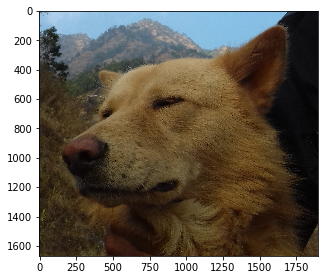## Circle

Everyone knows what is circle but only few cares how did it originated? Thanks to Euclid and his contribution to the modern Mathematics. Circle on simple term can be thought of as a shape where infinite points are present and the distance between two consecutive two points is infinitesimally small. The Computer Graphics doesn’t cares about that what it needs is a number. So if we zoom the shapes we start to see the pixels crystal clear. Here I will be using a simple concept of drawing a circle. I will be using the concept of polar form. If I have to write it on steps then:-

• Read an input image, get a radius for circle, get a center point, get a border, get a smoothness value and get a color value for it.
• Prepare smoothness * 360 angles for circle (of course 0 to 360).
• For each angle:
• Convert angle to radian from degree (NumPy geometrical functions takes radian).
• Find the distance between two points on circumference by Pythagoras theorem.
• Find the new point on circumference and make that point’s color on circle color if point is on first quadrant.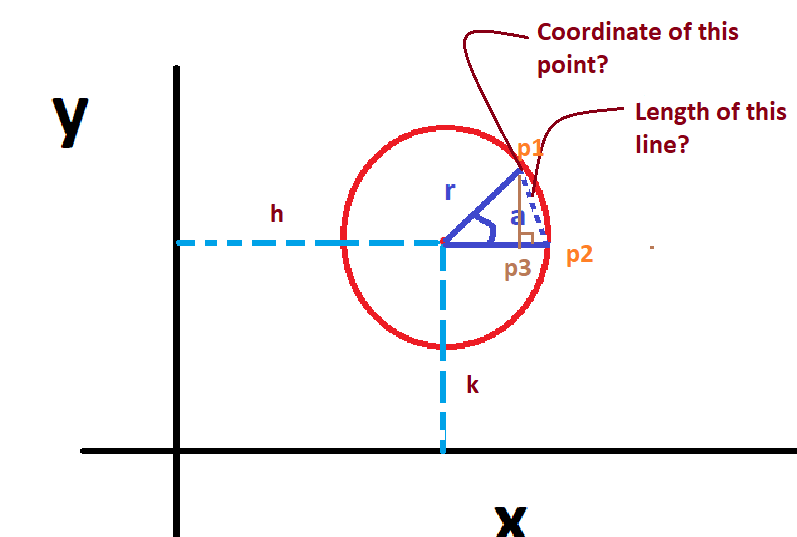Lets take an example of above circle on 2d plane. Circle’s center is on (h, k) its radius is r, and there are 2 points on circumference p1, p2, and third point is drawn on radius line joining p2 and center. Additionally, p3 is perpendicular to line joining p2 and center. Here we know length of 2 lines but not the line p1p3. But when the points p1 and p2 are so near that the distance between them is nearly zero (or limit tends to 0) then the point p3 will be at p2. At that time we can apply Pythagoras theorem. Below figure show a zoomed version of that situation.But what we need is the coordinate values of p1. We can do that by thinking as c is on origin. then the x coordinate of p1 will be equal to x coordinate of p3. And to find x-coordinate of p3 we can solve.

$cos(\theta) = \frac{b}{h}\\ x = b = cos(\theta) * h$

Similarly,

$sin(\theta) = \frac{p}{h}\\ y = p = sin(\theta) * h$

And on our case, when the circle is not on center then our (x, y) coordinate or p1 will be (h, k) far from plane’s center.

Hence, coordinate value for p1 will be:

$x = h + cos(\theta) * r\\ y = k + sin(\theta) * r$

And on image plane, the coordinate starts from (0, 0) and we don’t have -ve quadrant. Hence we ignore all (x, y) values that lies other than first quadrant. Enough of this theory, lets write that on code.

All the stories given above is already found on the polar form.

$x = cos(\theta) * r\\ y = sin(\theta) * r\\ and,\\ r = \sqrt{x^2 + y^2}\\ and,\\ \theta = tan^{-1}(\frac{y}{x})$
# creating a circle
def circle(img=None, center=(0, 0), rad=10, border=4, color=, smooth=2):
"""
A method to create a circle on a give image.
img: Expects numpy ndarray of image.
center: center of a circle
border: border of the circle, if -ve, circle is filled
color: color for circle
smooth: how smooth should our circle be?(smooth * 360 angles in 0 to 360)
"""
if type(img) == None:
raise ValueError("Image can not be None. Provide numpy array instead.")
angles = 360
cvalue = np.array(color)
if type(img) != type(None):
shape = img.shape
if len(shape) == 3:
row, col, channels = shape
else:
row, col = shape
channels = 1
angles = np.linspace(0, 360, 360*smooth)
for i in angles:
a = i*np.pi/180
y = center+rad*np.sin(a) # it is p=h*sin(theta)

# since we are wroking on image, coordinate starts from (0, 0) onwards and we have to ignore -ve values

if border >= 0:
b = int(np.ceil(border/2))

x1 = np.clip(x-b, 0, shape).astype(np.int32)
y1 = np.clip(y-b, 0, shape).astype(np.int32)
x2 = np.clip(x+b, 0, shape).astype(np.int32)
y2 = np.clip(y+b, 0, shape).astype(np.int32)

img[x1:x2, y1:y2] = cvalue

else:
x = np.clip(x, 0, shape)
y = np.clip(y, 0, shape)
r, c = int(x), int(y)

if i > 270:
img[center:r, c:center] = cvalue
elif i > 180:
img[r:center, c:center] = cvalue
elif i > 90:
img[r:center, center:c] = cvalue
elif i > 0:
img[center:r, center:c] = cvalue

return img

#ip.show(img)
fig = plt.figure(figsize=(5,5))
mimg = circle(img, center=(400, 100), border=20, rad=500)
ip.show(mimg)

<Figure size 360x360 with 0 Axes>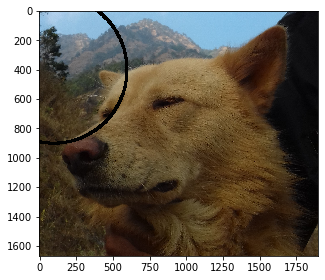Let me explain little bit of the code above.

• Check the inputs and loop for the angles.
• We will try to take as much as possible angles given by smoothness value.
• Take a coordinate value for a point to draw on.
• If the point lies on first quadrant:
• If the border value is +ve then draw only within the pixel and border/2 neighbor pixels on each of 4 directions.
• Else:
• If angle is greater than 270 then fill 4th quadrant with color
• If angle is greater than 190 then fill 3rd quadrant with color
• If angle is greater than 90 then fill 2nd quadrant with color
• If angle is greater than 0 then fill 1st quadrant with color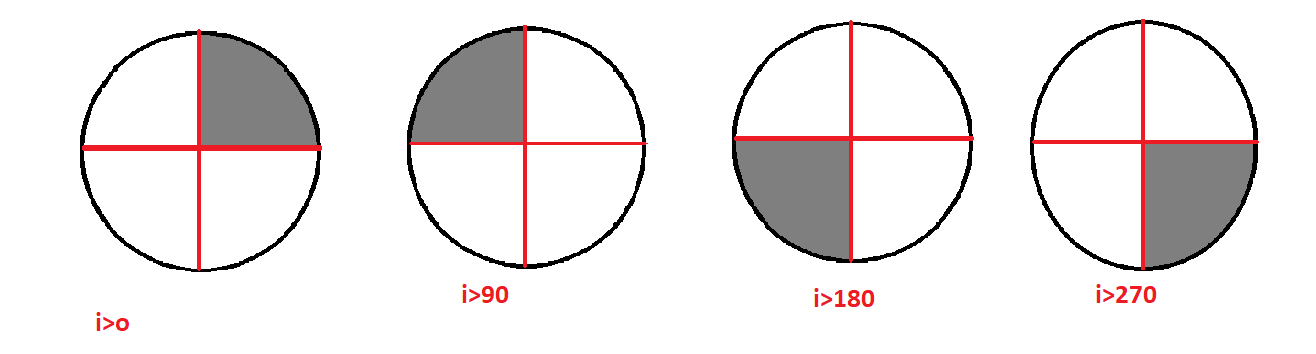### Compare it with OpenCV’s Circle

Before comparing with OpenCV, lets have a clear understanding of 2d graph plane and image plane. Image plane starts from the top left side but 2d graph plane starts from the center to upwards. Hence in order to compare our circle, we have to change the center value (in this case).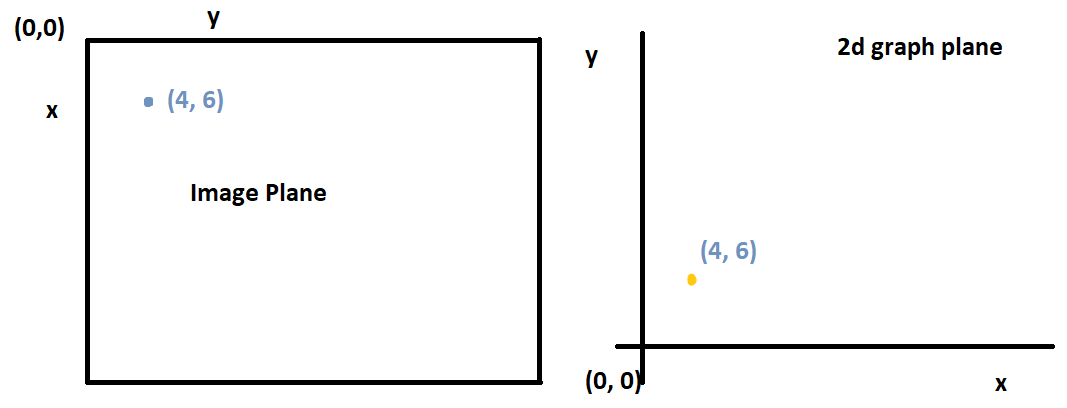And on this case, I am just swapping center values (i.e. (x, y) for OpenCV and (y, x) for ours).

• Draw circle on image using OpenCV
• Draw circle on same image using our method.
• Subtract drawn images
• Show the difference.

The common parts on images are shown complete black and those which are not are shown comlete white.

# read image
# draw using opencv
print("OpenCV")
cimg = cv2.circle(img.copy(), (400, 1000), 500, [0, 0, 0], -20)
ip.show(cimg)

# draw using our method (swap center)
print("Ours")
mimg = circle(img, center=(1000, 400), border=-20, rad=500, color=[0, 0, 0])
ip.show(mimg)

# difference
print("Difference")
r = mimg-cimg
r[r!=[0, 0, 0]] = 255
ip.show(r)

# count difference pixels
diff = np.sum(mimg!=cimg)
shape = mimg.shape

# what percentage is different?
diff * 100 / (shape * shape)

OpenCV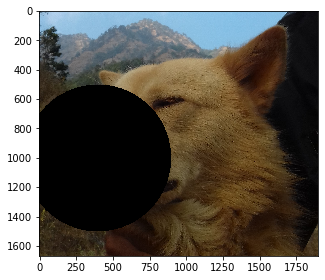Ours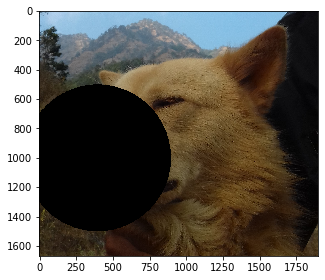Difference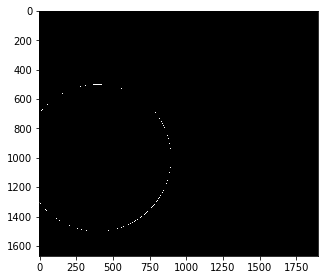0.2912136361400096


It seems clear that only 0.29% of pixels were different than the result of OpenCV’s circle. But the difference varies with the shape of circle.

# read image
# draw using opencv
print("OpenCV")
cimg = cv2.circle(img.copy(), (900, 1000), 500, [0, 0, 0], 20)
ip.show(cimg)

# draw using our method (swap center)
print("Ours")
mimg = circle(img.copy(), center=(1000, 900), border=20, rad=500, color=[0, 0, 0])
ip.show(mimg)

# difference
print("Difference")
r = mimg-cimg
r[r!=[0, 0, 0]] = 255
ip.show(r)

# count difference pixels
diff = np.sum(mimg!=cimg)
shape = mimg.shape

# what percentage is different?
diff * 100 / (shape * shape)

OpenCV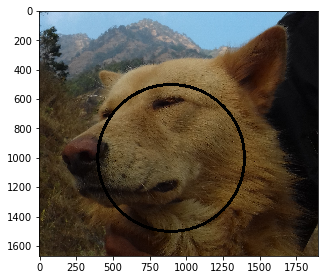Ours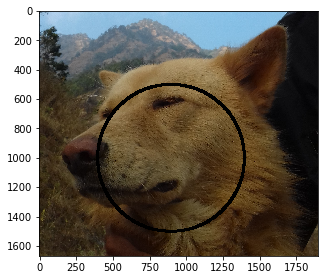Difference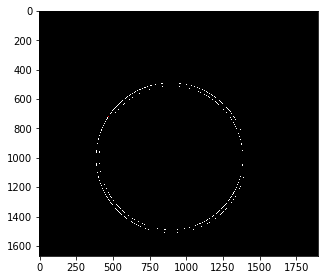1.064850381662056


## Rectangle

Drawing a Rectangle is very easy, in fact just an array indexing completes the task. We need coordinates of two opposite corners i.e. major diagonal. Top left and bottom right corner’s coordinate is required on this case. And we will perform array indexing. Same as on above case, we will work with border and color values.

def rectangle(img, pt1, pt2, border=2, color=):
"""
img: Input image where we want to draw rectangle:
pt1: top left point (y, x)
pt2: bottom right point
border: border of line
color: color of rectangle line,
returns new image with rectangle.

"""
p1 = pt1
pt1 = (p1, p1)
p2 = pt2
pt2 = (p2, p2)
b = int(np.ceil(border/2))
cvalue = np.array(color)
if border >= 0:
# get x coordinates for each line(top, bottom) of each side
# if -ve coordinates comes, then make that 0
x11 = np.clip(pt1-b, 0, pt2)
x12 = np.clip(pt1+b+1, 0, pt2)
x21 = pt2-b
x22 = pt2+b+1

y11 = np.clip(pt1-b, 0, pt2)
y12 = np.clip(pt1+b+1, 0, pt2)
y21 = pt2-b
y22 = pt2+b+1
# right line
img[x11:x22, y11:y12] = cvalue
#left line
img[x11:x22, y21:y22] = cvalue
# top line
img[x11:x12, y11:y22] = cvalue
# bottom line
img[x21:x22, y11:y22] = cvalue

else:
pt1 = np.clip(pt1, 0, pt2)
img[pt1:pt2+1, pt1:pt2+1] = cvalue

return img

mimg = rectangle(img, (100,500), (1000, 1000), border=-5, color=[20, 150, 20])
ip.show(mimg)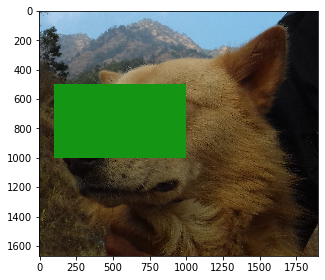Lets explain little bit of the code here:-

• Take an image where we want to draw, take coordinates of corners, take border of rectangle, take color of rectangle.
• Extract the coordinates where we want to draw (if the coordinates is out of image plane then perform clipping)
• If border is +ve:
• Change pixels on topmost line(top coordinate along with its some neighbors)
• Change pixels on bottommost line(top coordinate along with its some neighbors)
• Follow same for other lines.
• Else:
• Fill/Change color from top line to bottom from left to right line.

## Compare with OpenCV

# read image
# draw using opencv
print("OpenCV")
cimg = cv2.rectangle(img.copy(), (100, 500), (1000, 1000), [0, 0, 0], -5)
ip.show(cimg)

# draw using our method (swap center)
print("Ours")
mimg = rectangle(img, (100,500), (1000, 1000), border=-5, color=[0, 0, 0])
ip.show(mimg)

# difference
print("Difference")
r = mimg-cimg
r[r!=[0, 0, 0]] = 255
ip.show(r)

# count difference pixels
diff = np.sum(mimg!=cimg)
shape = mimg.shape

# what percentage is different?
diff * 100 / (shape * shape)

OpenCV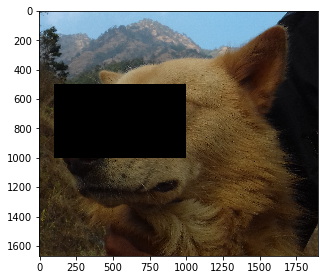OursDifference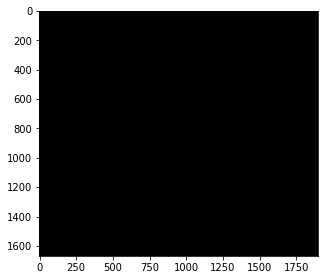0.0


The comparison with OpenCV seems to be great because we have 0 difference. You can try with different sizes of rectangle.

## Ellipse

Ellipse is a modified version of circle but it is well described as the portion that lies on a 2d plane when a plane is inclined inside a cone. Please search about this to see the bunch of images. I will again be using the polar form of ellipse. It is just as simple as the circle’s except we use axis instead of radius.

$x = h + cos(\theta) * a\\ y = k + sin(\theta) * b$

A simple example can be done using Matplotlib’s plot.

h = 2
k = 1
a = 3
b = 1

t = np.linspace(0, 2 * np.pi, 100)
plt.plot(h+a*np.cos(t), k+b*np.sin(t))
plt.plot()

[]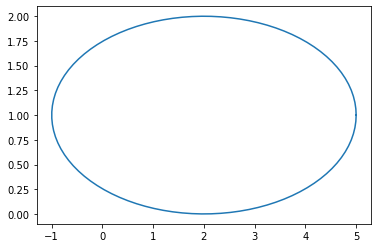# creating a ellipse
def ellipse(img=None, center=(0, 0), a=3, b=1, border=4, color=, smooth=2):
"""
A method to create a ellipse on a give image.
img: Expects numpy ndarray of image.
center: center of a ellipse
a: major axis
b: minor axis
border: border of the ellipse, if -ve, ellipse is filled
color: color for ellipse
smooth: how smooth should our ellipse be?(smooth * 360 angles in 0 to 360)
"""
if type(img) == None:
raise ValueError("Image can not be None. Provide numpy array instead.")
angles = 360
cvalue = np.array(color)
if type(img) != type(None):
shape = img.shape
if len(shape) == 3:
row, col, channels = shape
else:
row, col = shape
channels = 1
angles = np.linspace(0, 360, 360*smooth)
for i in angles:
angle = i*np.pi/180
y = center+b*np.sin(angle)
x = center+a*np.cos(angle)

# since we are wroking on image, coordinate starts from (0, 0) onwards and we have to ignore -ve values
if border >= 0:
r, c = int(x), int(y)
bord = int(np.ceil(border/2))
x1 = np.clip(x-bord, 0, img.shape).astype(np.int32)
y1 = np.clip(y-bord, 0, img.shape).astype(np.int32)
x2 = np.clip(x+bord, 0, img.shape).astype(np.int32)
y2 = np.clip(y+bord, 0, img.shape).astype(np.int32)

img[x1:x2, y1:y2] = cvalue

else:
x = np.clip(x, 0, img.shape)
y = np.clip(y, 0, img.shape)
r, c = int(x), int(y)
if i > 270:
img[center:r, c:center] = cvalue
elif i > 180:
img[r:center, c:center] = cvalue
elif i > 90:
img[r:center, center:c] = cvalue
elif i > 0:
img[center:r, center:c] = cvalue

return img

mimg = np.zeros((100, 100, 3), dtype=np.int32) + 255
eimg = ellipse(mimg.copy(), center=(10, 30), a = 10, b = 40, border=-2, color=[0, 0, 0])
ip.show(eimg)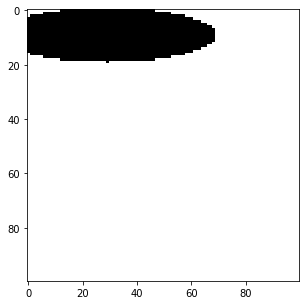## Compare it with OpenCV’s Ellipse

Again, the case is just like circle’s, we have to swap center and the axes for the ellipse.

cimg = cv2.ellipse(mimg.copy(), (30, 10), (40, 10), 0, 0, 360, [0, 0, 0], -2)
ip.show(cimg)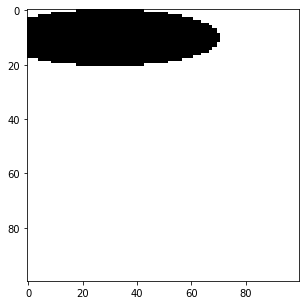# difference on fill
diff = np.sum(cimg!=eimg)
shape = cimg.shape

# what percentage is different?
diff * 100 / (shape * shape)

4.47

# difference on normal
mimg = np.zeros((100, 100, 3), dtype=np.int32) + 255
eimg = ellipse(mimg.copy(), center=(20, 30), a = 10, b = 40, border=2, color=[0, 0, 0])
print("Ours")
ip.show(eimg)

# opencv's
print("OpenCV's")
cimg = cv2.ellipse(mimg.copy(), (30, 20), (40, 10), 0, 0, 360, [0, 0, 0], 2)
ip.show(cimg)

# difference on fill
diff = np.sum(cimg!=eimg)
shape = cimg.shape

# what percentage is different?
diff * 100 / (shape * shape)

Ours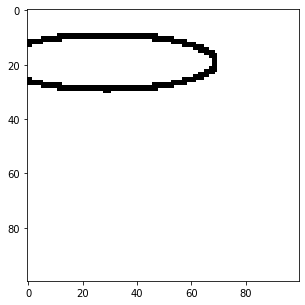OpenCV's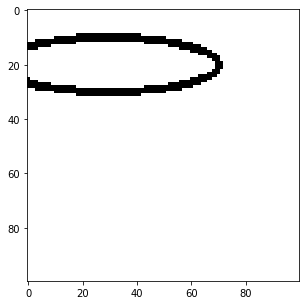8.55


The difference of OpenCV’s and our method’s output is not that bad. But as always, the difference depends on the size of the shape.

## Finally

We have written simple methods to perform basic geometric shapes drawing. Now on bonus topic I will add these methods on our Image Processing class.

## Bonus Topic

class ImageProcessing:
def __init__(self):
self.readmode = {1 : "RGB", 0 : "Grayscale"}

def read_image(self, location = "", mode = 1):
"""
Uses imageio on back.
* location: Directory of image file.
* mode: Image readmode{1 : RGB, 0 : Grayscale}.
"""

if mode == 1:
img = img
elif mode == 0:
img = self.convert_color(img, 0)
elif mode == 2:
pass
else:
return img

def show(self, image, figsize=(5, 5)):
"""
Uses Matplotlib.pyplot.
* image: A image to be shown.
* figsize: How big image to show. From plt.figure()

"""
fig = plt.figure(figsize=figsize)
im = image
plt.imshow(im, cmap='gray')
plt.show()

def convert_color(self, img, to=0):
if to==0:
return  0.21 * img[:,:,0] + 0.72 * img[:,:,1] + 0.07 * img[:,:,2]
else:
raise ValueError("Color conversion can not understood.")

# creating a circle
def circle(self, img=None, center=(0, 0), rad=10, border=4, color=, smooth=2):
"""
A method to create a circle on a give image.
img: Expects numpy ndarray of image.
center: center of a circle
border: border of the circle, if -ve, circle is filled
color: color for circle
smooth: how smooth should our circle be?(smooth * 360 angles in 0 to 360)
"""
if type(img) == None:
raise ValueError("Image can not be None. Provide numpy array instead.")
angles = 360
cvalue = np.array(color)
if type(img) != type(None):
shape = img.shape
if len(shape) == 3:
row, col, channels = shape
else:
row, col = shape
channels = 1
angles = np.linspace(0, 360, 360*smooth)
for i in angles:
a = i*np.pi/180
y = center+rad*np.sin(a) # it is p=h*sin(theta)

# since we are wroking on image, coordinate starts from (0, 0) onwards and we have to ignore -ve values

if border >= 0:
b = int(np.ceil(border/2))

x1 = np.clip(x-b, 0, shape).astype(np.int32)
y1 = np.clip(y-b, 0, shape).astype(np.int32)
x2 = np.clip(x+b, 0, shape).astype(np.int32)
y2 = np.clip(y+b, 0, shape).astype(np.int32)

img[x1:x2, y1:y2] = cvalue

else:
x = np.clip(x, 0, shape)
y = np.clip(y, 0, shape)
r, c = int(x), int(y)

if i > 270:
img[center:r, c:center] = cvalue
elif i > 180:
img[r:center, c:center] = cvalue
elif i > 90:
img[r:center, center:c] = cvalue
elif i > 0:
img[center:r, center:c] = cvalue

return img

def rectangle(self, img, pt1, pt2, border=2, color=):
"""
img: Input image where we want to draw rectangle:
pt1: top left point (y, x)
pt2: bottom right point
border: border of line
color: color of rectangle line,
returns new image with rectangle.

"""
p1 = pt1
pt1 = (p1, p1)
p2 = pt2
pt2 = (p2, p2)
b = int(np.ceil(border/2))
cvalue = np.array(color)
if border >= 0:
# get x coordinates for each line(top, bottom) of each side
# if -ve coordinates comes, then make that 0
x11 = np.clip(pt1-b, 0, pt2)
x12 = np.clip(pt1+b+1, 0, pt2)
x21 = pt2-b
x22 = pt2+b+1

y11 = np.clip(pt1-b, 0, pt2)
y12 = np.clip(pt1+b+1, 0, pt2)
y21 = pt2-b
y22 = pt2+b+1
# right line
img[x11:x22, y11:y12] = cvalue
#left line
img[x11:x22, y21:y22] = cvalue
# top line
img[x11:x12, y11:y22] = cvalue
# bottom line
img[x21:x22, y11:y22] = cvalue

else:
pt1 = np.clip(pt1, 0, pt2)
img[pt1:pt2+1, pt1:pt2+1] = cvalue

return img

# creating a ellipse
def ellipse(self, img=None, center=(0, 0), a=3, b=1, border=4, color=, smooth=2):
"""
A method to create a ellipse on a give image.
img: Expects numpy ndarray of image.
center: center of a ellipse
a: major axis
b: minor axis
border: border of the ellipse, if -ve, ellipse is filled
color: color for ellipse
smooth: how smooth should our ellipse be?(smooth * 360 angles in 0 to 360)
"""
if type(img) == None:
raise ValueError("Image can not be None. Provide numpy array instead.")
angles = 360
cvalue = np.array(color)
if type(img) != type(None):
shape = img.shape
if len(shape) == 3:
row, col, channels = shape
else:
row, col = shape
channels = 1
angles = np.linspace(0, 360, 360*smooth)
for i in angles:
angle = i*np.pi/180
y = center+b*np.sin(angle)
x = center+a*np.cos(angle)

# since we are wroking on image, coordinate starts from (0, 0) onwards and we have to ignore -ve values
if border >= 0:
r, c = int(x), int(y)
bord = int(np.ceil(border/2))
x1 = np.clip(x-bord, 0, img.shape).astype(np.int32)
y1 = np.clip(y-bord, 0, img.shape).astype(np.int32)
x2 = np.clip(x+bord, 0, img.shape).astype(np.int32)
y2 = np.clip(y+bord, 0, img.shape).astype(np.int32)

img[x1:x2, y1:y2] = cvalue

else:
x = np.clip(x, 0, img.shape)
y = np.clip(y, 0, img.shape)
r, c = int(x), int(y)
if i > 270:
img[center:r, c:center] = cvalue
elif i > 180:
img[r:center, c:center] = cvalue
elif i > 90:
img[r:center, center:c] = cvalue
elif i > 0:
img[center:r, center:c] = cvalue

return img

def convolve(self, image, kernel = None, padding = "zero", stride=(1, 1), show=False, bias = 0):
"""
* image: A image to be convolved.
* kernel: A filter/window of odd shape for convolution. Used Sobel(3, 3) default.
* padding: Border operation. Available from zero, same, none.
* stride: How frequently do convolution?
"""

if len(image.shape) > 3:
raise ValueError("Only 2 and 3 channel image supported.")
if type(kernel) == type(None):
warnings.warn("No kernel provided, trying to apply Sobel(3, 3).")
kernel = np.array([[-1, 0, 1],
[-1, 0, 1],
[-1, 0, 1]])
kernel += kernel.T
kshape = kernel.shape
if kshape % 2 != 1 or kshape % 2 != 1:
raise ValueError("Please provide odd length of 2d kernel.")

if type(stride) == int:
stride = (stride, stride)

shape = image.shape

zeros_h = np.zeros(shape).reshape(-1, shape)
zeros_v = np.zeros(shape+2).reshape(shape+2, -1)

shape = image.shape

h1 = image.reshape(-1, shape)
h2 = image[-1].reshape(-1, shape)

shape = image.shape
pass

rv = 0
cimg = []
for r in range(kshape, shape+1, stride):
cv = 0
for c in range(kshape, shape+1, stride):
chunk = image[rv:r, cv:c]
soma = (np.multiply(chunk, kernel)+bias).sum()
try:
chunk = int(soma)
except:
chunk = int(0)
if chunk < 0:
chunk = 0
if chunk > 255:
chunk = 255
cimg.append(chunk)
cv+=stride
rv+=stride
cimg = np.array(cimg, dtype=np.uint8).reshape(int(rv/stride), int(cv/stride))
if show:
return cimg
ip = ImageProcessing()
cv = ip.convolve(img)
ip.show(cv)

#ip.show(img)
fig = plt.figure(figsize=(5,5))
mimg = ip.circle(img, center=(400, 100), border=20, rad=500)
ip.show(mimg)

mimg = ip.rectangle(img, (100,500), (1000, 1000), border=-5, color=[20, 150, 20])
ip.show(mimg)

mimg = np.zeros((100, 100, 3), dtype=np.int32) + 255
eimg = ip.ellipse(mimg.copy(), center=(10, 30), a = 10, b = 40, border=-2, color=[0, 0, 0])
ip.show(eimg)


C:\ProgramData\Anaconda3\lib\site-packages\ipykernel_launcher.py:211: UserWarning: No kernel provided, trying to apply Sobel(3, 3).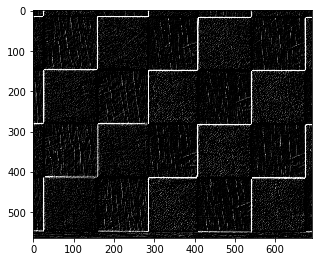<Figure size 360x360 with 0 Axes>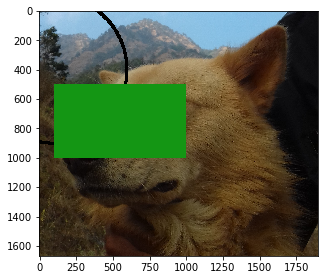Thank you so much for reading this and if you find it interesting why not share it or leave the comments? If you have any queries then you can send me mail or find me at Twitter as @QuassarianViper.

## What next?

• Add functionality to do blurring, noise cancellation, sharpening etc
• Add functionality to do erosion, dilation etc operations.

In the meantime how about looking over some of mine works?